I can teach you Snowflake analytics!  Today we are going to learn about Cume_Dist.

Cume_Dist returns the cumulative distribution of a value within a set of values. It represents the number of rows with values less than or equal to that row’s value divided by the total number of rows. The returned value of the CUME_DIST function is greater than zero and less than or equal one.  Check out the example below.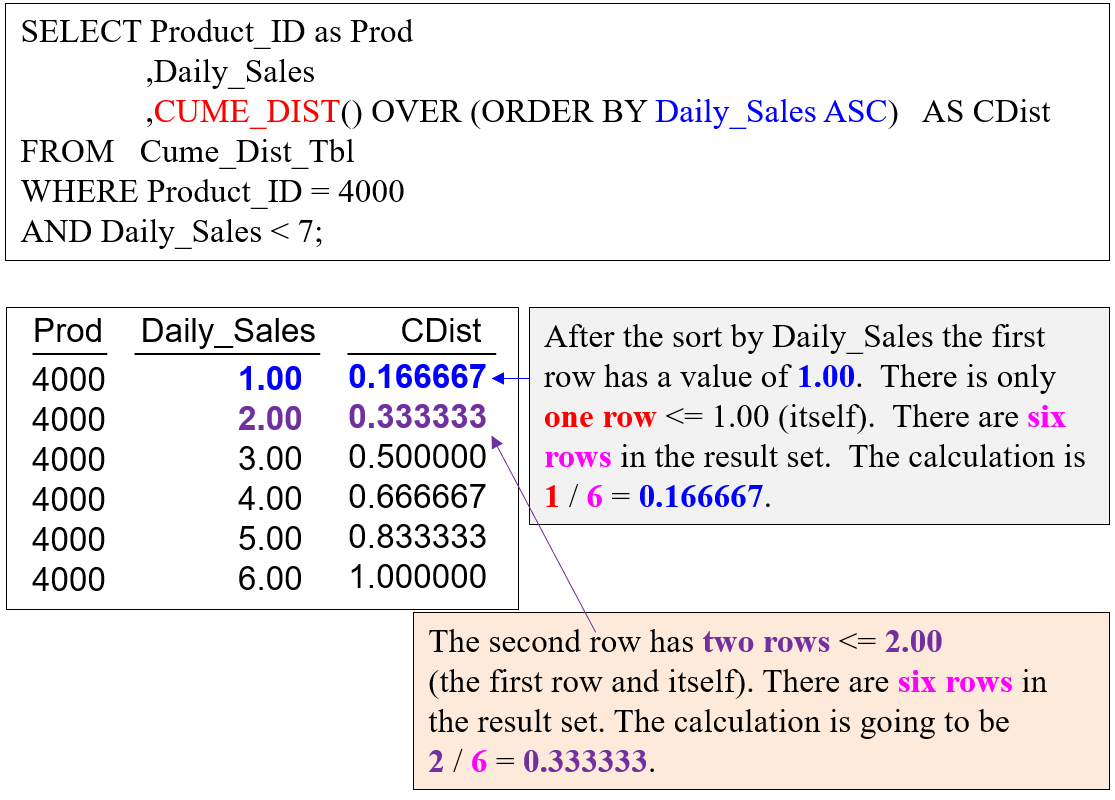The next example is similar, but it uses a QUALIFY statement.  The QUALIFY statement is to ordered analytics what the HAVING statement is to aggregation.  QUALIFY and HAVING are different than a WHERE clause filter.  A WHERE clause filters a row out before any calculations take place.  QUALIFY waits until the row calculations take place, and then it acts as a further filter on the totals.  As an example, if you have a WHERE clause and a QUALIFY, like an example below, the WHERE only calculates rows that have a Product_ID = 4000 AND Daily_Sales < 7.  Once the calculations happen, the QUALIFY further eliminates rows whose calculations do not meet the criteria.The next example merely shows more rows to give you a better idea of the calculations.  I want you to realize that if the last value of Daily_Sales were a billion, it would not matter that its total was so far greater than the others.  The Cume_Dist calculation would merely ask when the ORDER BY is in ASC mode, “How many rows have a Daily_Sales value less than or equal to you?”  The Cume_Dist will then divide that number by the total number of rows in the window.

It is the opposite when the ORDER BY is in DESC mode.  The Cume_Dist calculation will ask, “How many rows have a Daily_Sales value greater than or equal to you?”  The Cume_Dist will then divide that number by the total number of rows in the window.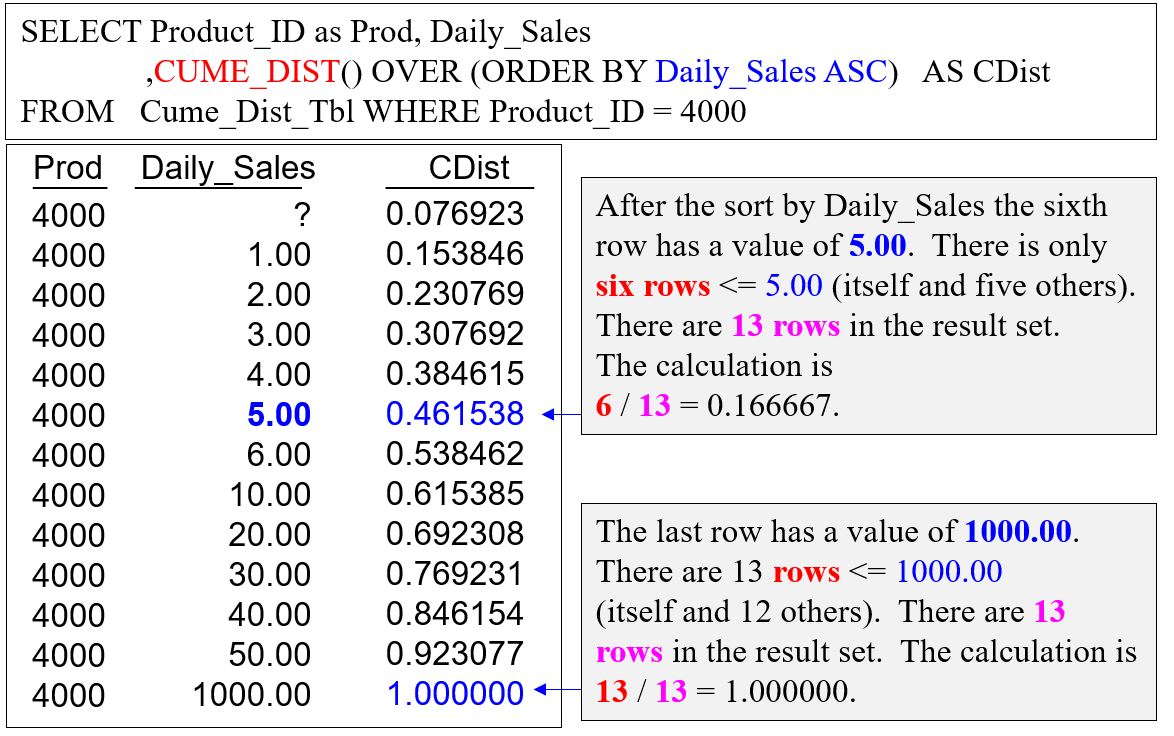The next example shows you how Cume_Dist calculates when there are equal values among the rows.  We have multiple Daily_Sales values that are equal (tie).  Notice that our ORDER BY is in DESC mode.  Once again, the Cume_Dist merely asks, “How many rows are greater than or equal to your Daily_Sales?”  It will then divide that number by the total number of rows.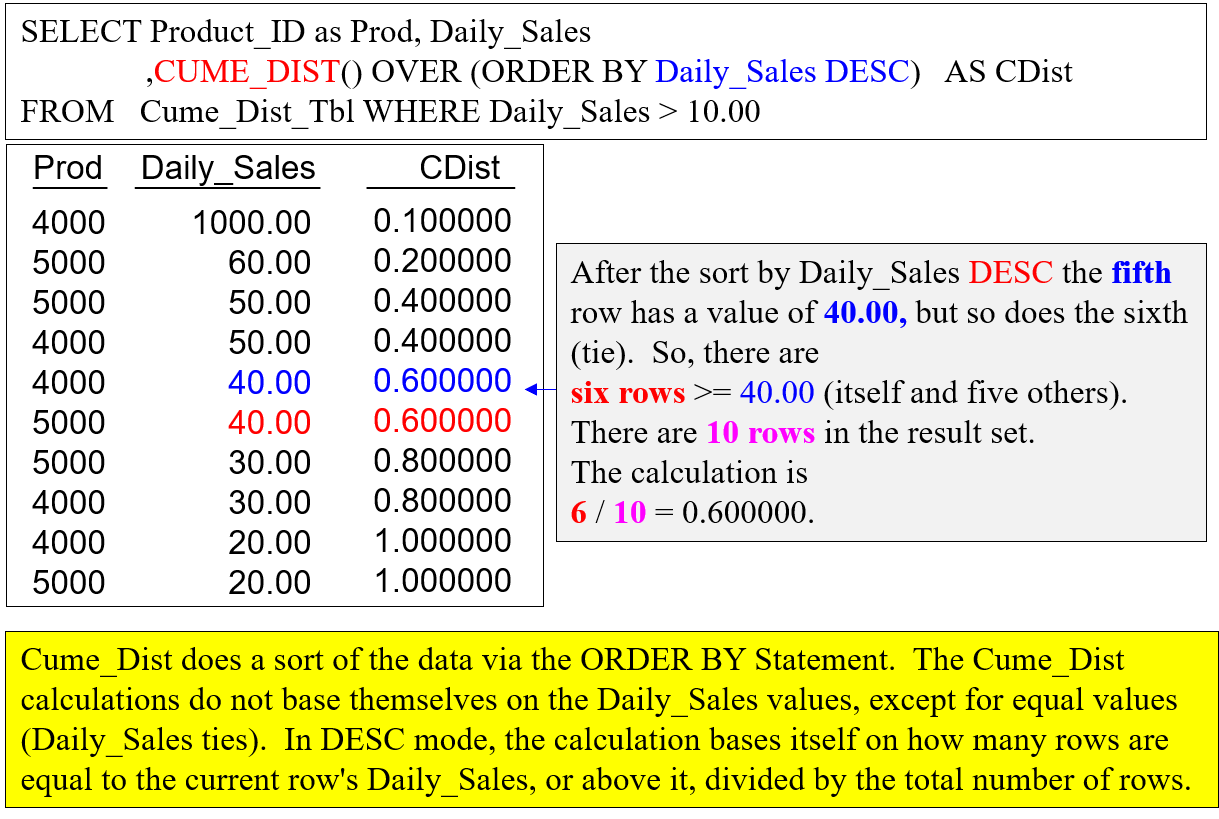Next, we are going to take the next example even farther by adding a PARTITION BY Clause.  PARTITION BY resets the Cume_Dist calculation with each Product_ID break. That means the calculations for Product_ID 4000 only calculate based on rows with a Product_ID of 4000.  The calculations for Product_ID 5000 only calculate based on rows with a Product_ID of 5000.

What is interesting here is how the data sorts.  Let me run you through this in slow motion.

The first Cume_Dist calculation does not have PARTITION BY, and it orders the data by Daily_Sales DESC.  Then, the calculation takes place for only the column with the alias CDist.

Then, the Cume_Dist calculation happens for the column with the alias CDist_Partition.  The data sort first by the partition of Product_ID, and then sorts by Daily_Sales DESC.  Then, the calculation takes place for only the column with the alias CDist_Partition.

However, the final report is in the order of the last Cume_Dist calculation.  Therefore, the column CDist looks like it is out of sort, but the Daily_Sales that are equal (tied), have the same Cume_Dist.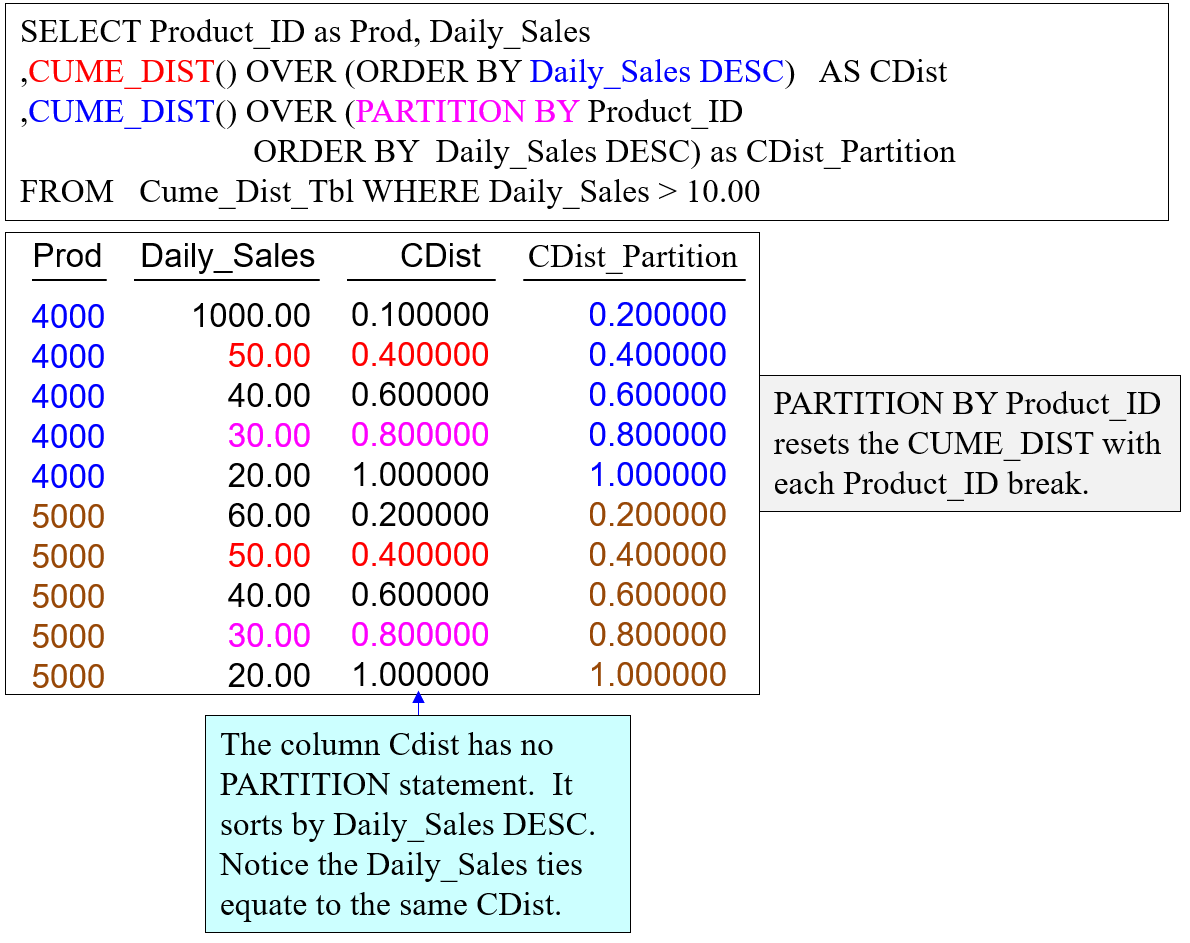One of the great features of Cume_Dist is how easy it is to chart.  You should be able to point-and-click and graph/chart every answer set. How would you like to use a tool that does this and automatically places charts in a dashboard?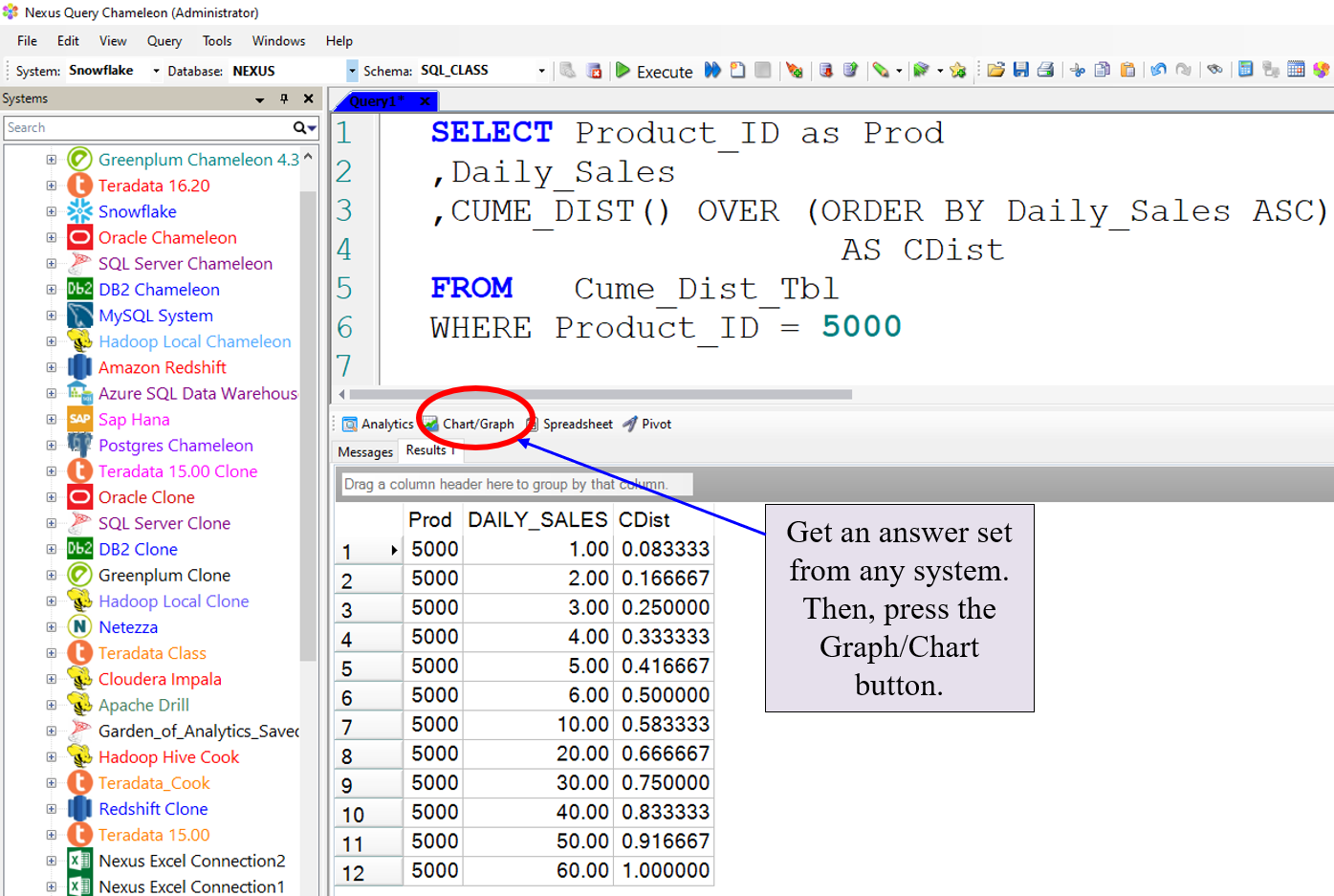Once you hit the Graph/Chart button, the answer set is ready to become a graph or chart.  Now, use the Nexus Garden of Analytics by clicking on the template arrows to choose your columns for the Y-Axis, X-Axis, Sort By, and Partition By areas.  Then, choose your chart options and your visual type.  Hit CREATE and your chart instantly appears.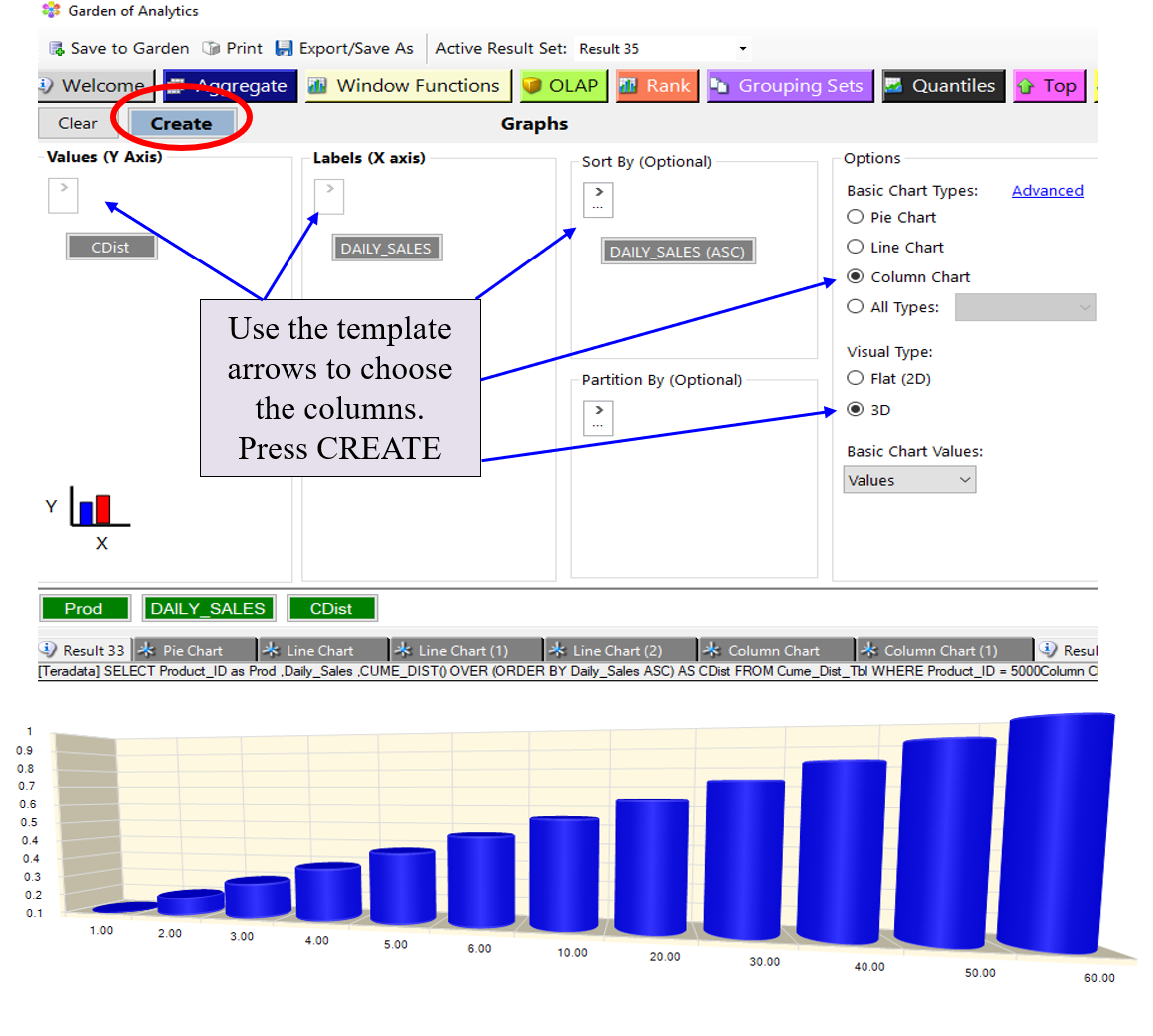I hope you enjoyed today’s Snowflake analytic lesson.  See you next week.

Thank you,

Tera-Tom

Tom Coffing

CEO, Coffing Data Warehousing

Direct: 513 300-0341

Website: www.CoffingDW.com